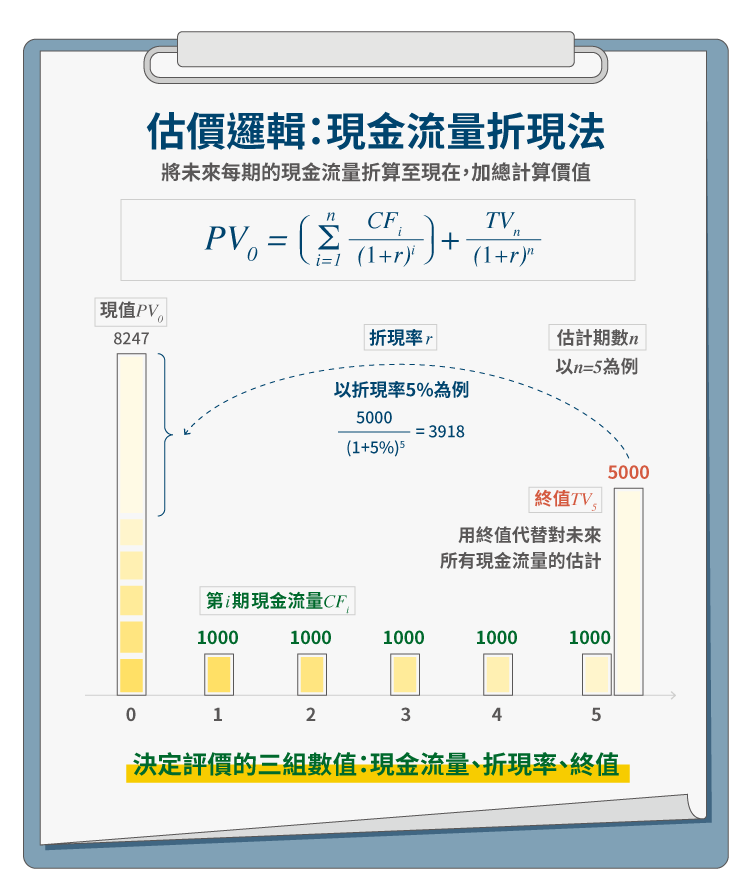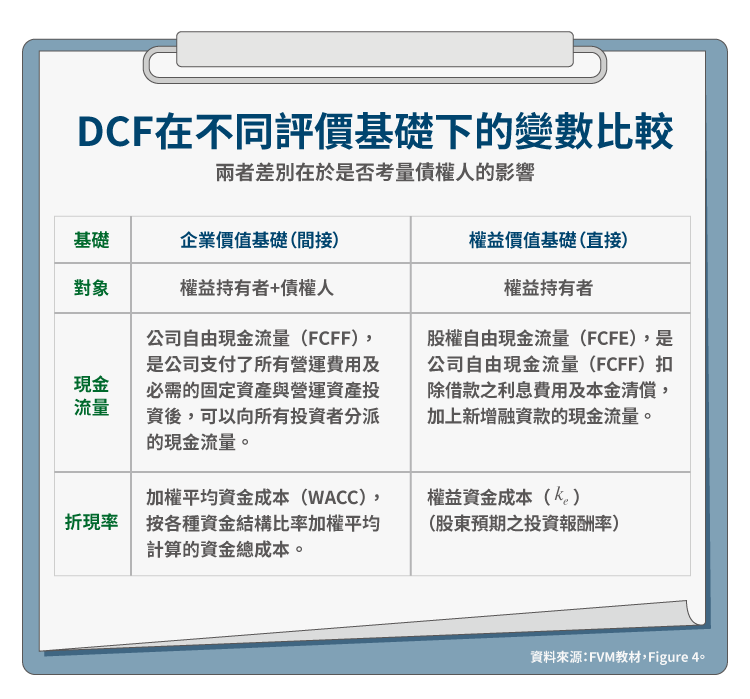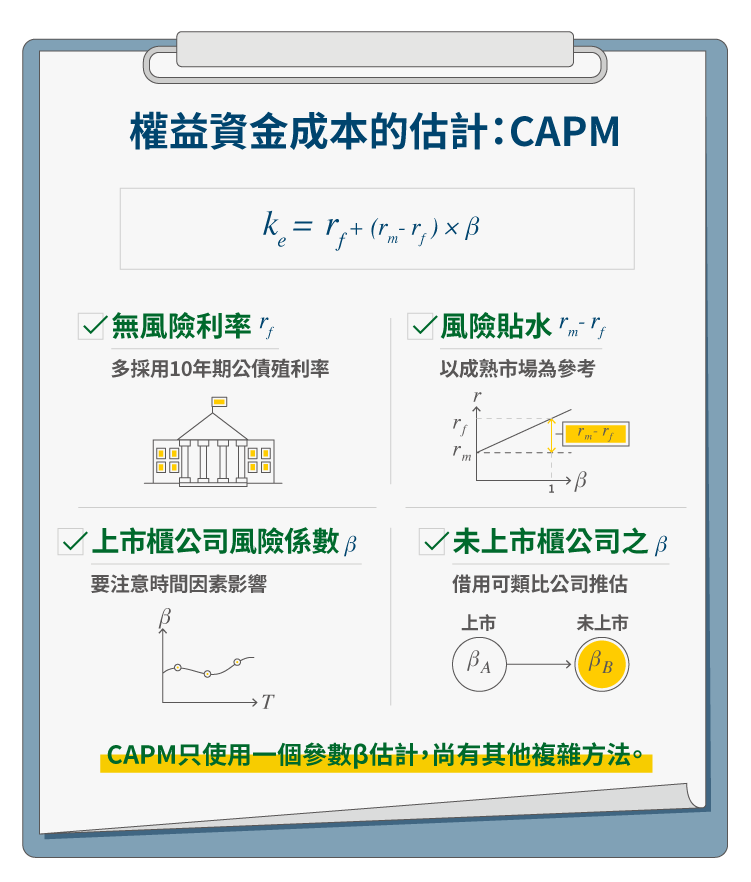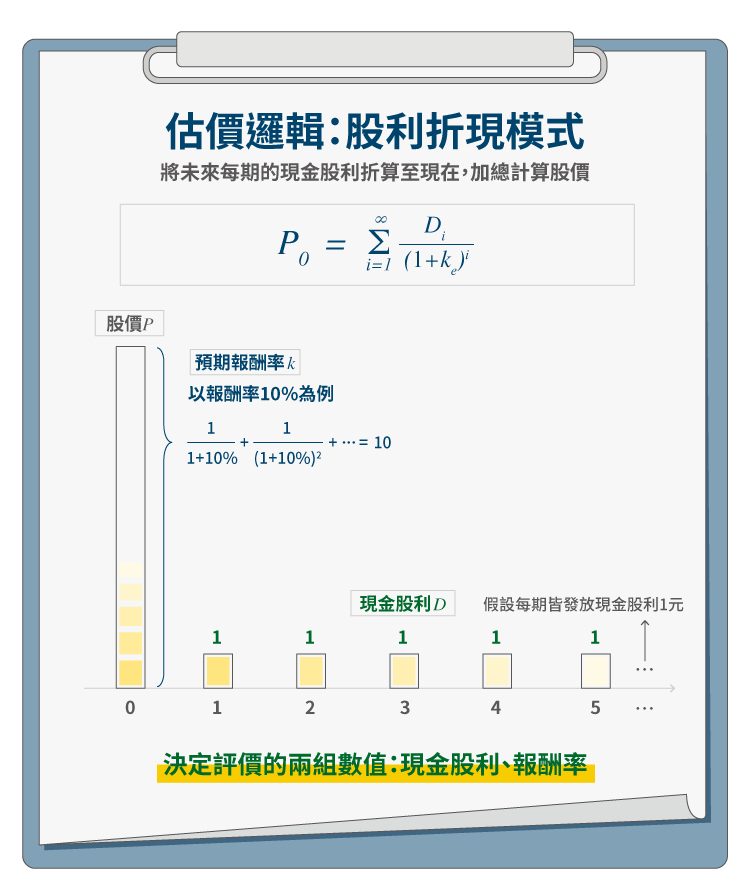台灣經濟新報(TEJ)

# 未上市股權評價：收益法

2019 年 2 月 21 日

FVM 教材對收益法之指引，以「現金流量折現法（Discounted Cash Flow method, DCF）」為核心，兼及「股利折現模式（Dividend Discount Model, DDM）」，與兩個對應的簡化模式：固定成長 DCF―資本化模式（Capitalization model）、固定成長 DDM（Constant-growth DDM）。

## 現金流量折現法DCF 模式的基本技術，就是折現：現值公式為 $$PV_0=(\sum\limits_{i=1}^n\frac{CF_i}{(1+r)^i})+\frac{TV_n}{(1+r)^n}$$。只要決定三組數值，就能完成評價：現金流量（Cash Flow, CF）、折現率（r）、終值（Terminal Value, TV）。其中因為我們不可能預期公司每年的現金流量，但公司又是假設永續經營存在，在此前提下，理論上應該對永續的現金流量做折現。$$FCFF=EBIT\cdot (1-t)+$$ 折舊及攤銷 $$-(RR+$$ 淨營運資金增額 $$)$$

$$FCFE=FCFF-$$ 利息費用 $$\cdot (1-t)-$$ 本金償還 $$+$$ 新增融資款

$$WACC=\frac{D}{D+E}\cdot (1-t)\cdot k_d+\frac{D}{D+E}\cdot k_e$$

$$D$$：債務之公允價值
$$E$$：股東權益之公允價值
$$k_d$$：債務資金成本
$$k_e$$：權益資金成本
$$t$$：企業所得稅稅率

$$EV_{op}=\sum\limits_{i=1}^n \frac{FCFF_i}{(1+WACC)^i}+\frac{1}{(1+WACC)^n}\cdot \frac{FCFF_{n+1}}{WACC-g}$$ ⋯（1）

$$EV=EV_{op}+$$ 非營運資產公允價值 − 非營運負債公允價值 ⋯（2）
$$E=EV-D$$ ⋯（3）

## 參數 2、權益之資金成本

FVM 教材，對於權益資金成本（$$k_e$$）的估計，僅介紹最傳統、最簡單的 CAPM。依 CAPM，權益的資金成本如下：

$$k_e=r_f+(r_m-r_f)\cdot\beta$$ （4）

$$r_f$$：無風險利率（risk-free rate），對無風險資產期望的報酬率
$$r_m$$：投資人要求的市場報酬率（required market rate of return），對一個充分風險分散之投資組合之預期報酬率
$$r_m-r_f$$：投資人要求的風險貼水（required equity premium）
$$\beta$$：單一資產的系統風險係數• （2-1）無風險利率：$$r_f$$

• （2-2）上市櫃公司風險係數：$$\beta$$

$$\beta$$ 應為前瞻性（forward-looking estimate），而非歷史 $$\beta$$。常見以歷史 $$\beta$$ 作為替代，所隱含的假設是：未來的波動與過去類似；換言之，若此假設不成立，就該進行前瞻性 $$\beta$$ 之估計。至於前瞻性 $$\beta$$ 如何估計，FVM 教材僅以選擇權市場帶過，實則極為複雜。

―若年度夠長，採月資料、週資料頻率即可（原則上，日資料雜訊過多）。一般來說，會採用過去 2～5 年的歷史資料推估 $$\beta$$；若僅用 2 年資料，多採週報酬；若估計期長達 5 年，就可能採月報酬。
―若市場波動過大，就會拉長期間，以免受短期波動之扭曲。

• （2-3）未上市櫃公司之 $$\beta$$

• （2-4）風險貼水：$$(r_m-r_f)$$

• （2-5）CAPM 之限制

CAPM 的基本假設是，個別股票風險及預期報酬與系統風險（systematic risk）呈線性相關。因其假設過簡，常受批評。其中的批評之一就是只有一個 $$\beta$$，風險涵蓋面太小，未納入其他重大風險考量，如規模大小、現金流量波動、流動性等。議題甚多，待有機會再行介紹。

## 固定成長 DCF―資本化模型

$$PV=\frac{FCFF}{WACC-g}=\frac{FCFF}{c}$$

## 股利折現模式$$P_0=\sum\limits_{i=1}^\infty \frac{D_i}{(1+k_e)^i}$$

$$P_0$$：評價日之評價結果
$$D_i$$：預期第 i 期發放之現金股利

$$D_i=D_{i-1}\cdot (1+g)=D_0\cdot (1+g)^i$$
$$P_0=\sum\limits_{i=1}^\infty \frac{D_0\cdot (1+g)^i}{(1+k_e)^i}=\frac{D_0\cdot (1+g)}{k_e-g}=\frac{D_1}{k_e-g}$$

## 結語

【延伸閱讀】台灣經濟新報(TEJ)